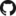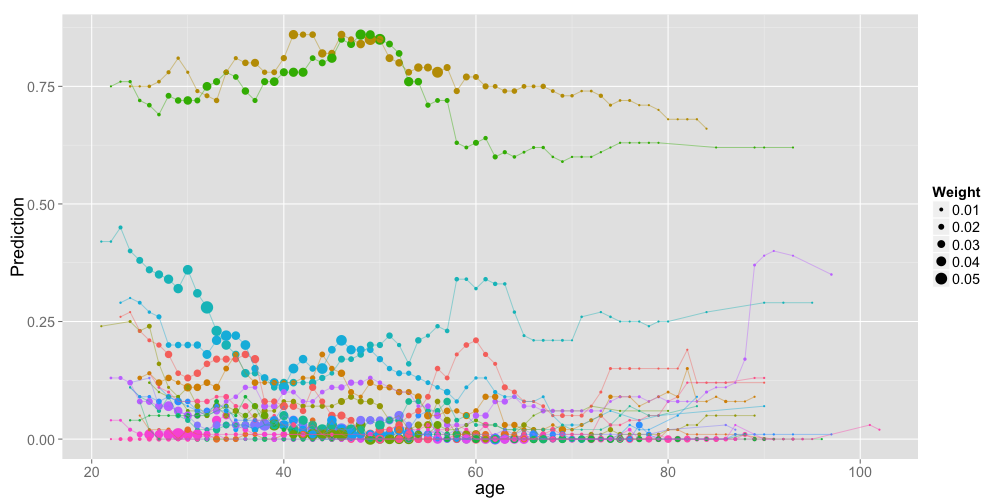# PredcompsHome

PDF Manual

Per Unit Input (original APC)

Impact (in units of output)

## Examples

Examples Overview

A fake logistic regression example predicting wine sales

A linear model with interactions

Loan defaults example

## More

As compared with Gelman & Pardoe 2007

Pairs & Weights

## As Compared With the Paper

There are a few differences between what's here and what's discribed in Gelman and Pardoe 2007. Many features described in the paper (such as categorical inputs) are just not implemented here. This page ignores those, and describes only outright differences, or additions.

### Pairs and Weights

This page comments on how the weights are computed and describes from differences between this package and what's described in section 4.1 of the paper.

### Absolute APCs

Gelman & Pardoe mention an unsigned version APCs in the case where the input of interest is an unordered categorical variable (in which case signs wouldn't make sense). They propose a root mean squared APC (equaton 4), but I prefer absolute values and I believe this absolute-values version is useful for any inputs, not just categorical ones. By default, I always compute and display an absolute version of the APC alongside the signed version. See e.g. the input $$u_8$$ in my simulated linear model with interactions for an artificial example demonstrating the importance of this notion, and see my explanation of APCs for more detail.

### Impact: A variation on APCs with comparable units

I've created a statistic similar to the original APC, but which addresses two issues I've had with APCs:

1. APCs are good for their purpose (the expected difference in outcome per unit change in input), but it doesn't tell me the what difference an input makes to my predictions. The APC could be high while the variation in the input is so small that it doesn't make a difference.

2. APCs across inputs with different units have different units themselves and so are not directly comparable. The example in the paper (see p. 47) uses mostly binary inputs, so this is mostly not a problem there. But I'm not sure the other inputs belong on the same chart.

Both (1) and (2) could be addressed by standardizing the coefficients before computing the APC, but this feels a bit ad hoc and arbitrary. Instead, I take the simpler and more elegant approach of just not dividing by the difference in inputs. The computed quantity is therefore the expected value of the predictive difference caused by a random transition for the input of interest. The units are the same as the output variable, and hence are always comparable across different inputs. Just as with APCs, this quantity depends on the model, the variation in the input of interest, and the relationship between that inputs and the other inputs.

I'm calling this notion impact (feel free to suggest another name), and it's described in more detail here and in the examples. Just like APCs, it comes in signed and absolute forms.

### All-else-equal Curves / Sensitivity Analysis

To visualize the model in more detail than is provided by our aggregated predictive comparisons, we can plot $$u$$ vs. the prediction at a variaty of values of $$v$$. In order that these plots represent $$p(u|v)$$, we can use the same set of pairs/weights as is constructed for computing aggregated predictive comparisons.

This plot shows age vs. probability of default, as in the loan defaults example:Plots like this are not yet computed in the package, but see the example for how to construct them.